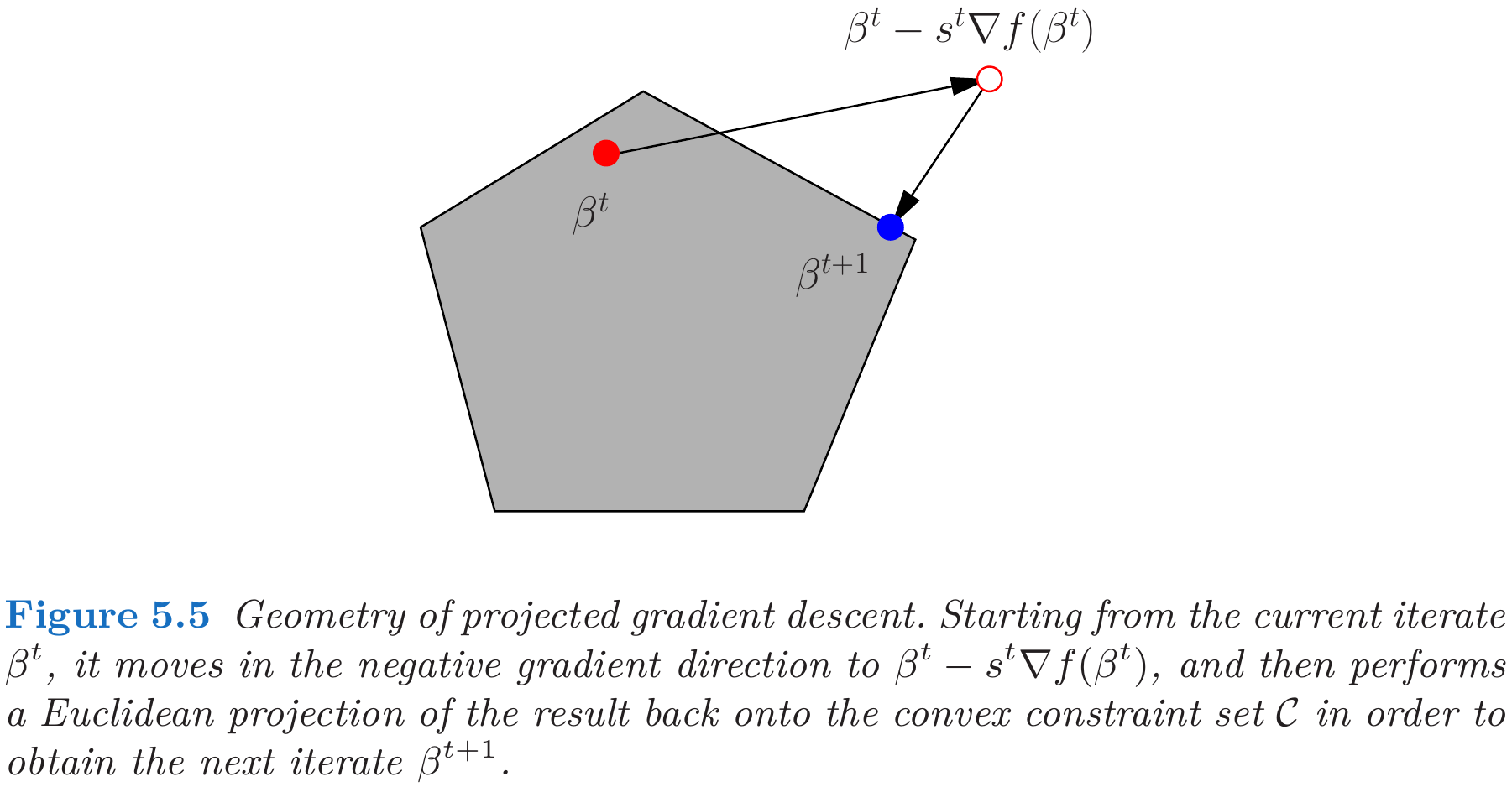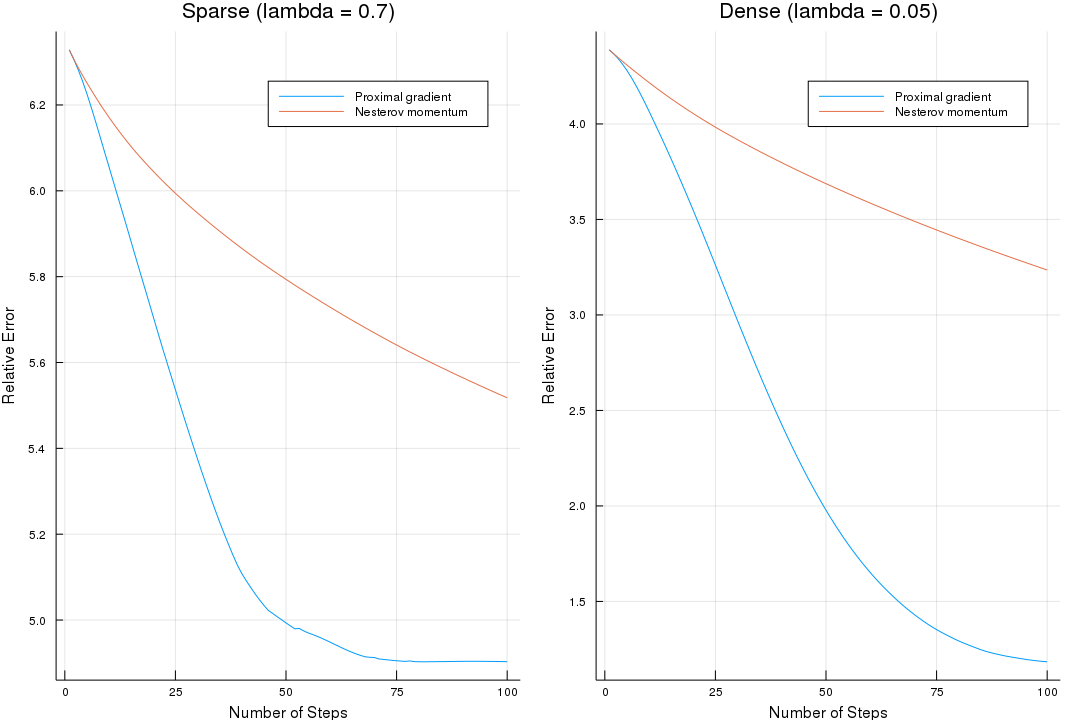# WeiYa's Work Yard

##### Posted on Mar 20, 2019 (Update: Apr 08, 2019)

I read the topic in kiytay’s blog: Proximal operators and generalized gradient descent, and then read its reference, Hastie et al. (2015), and write some program to get a better understanding.

Suppose we want to minimize a convex differentiable function $f:\bbR^p\rightarrow \bbR$, a common first-order iterative method is gradient descent,

$\beta^{t+1} = \beta^t - s^t\nabla f(\beta^t)\,,\quad \text{for }t=0,1,\ldots,$

which has the alternative representation

$\beta^{(t+1)} = \argmin_{\beta\in\bbR^p}\left\{f(\beta^t) + \langle \nabla f(\beta^t),\beta-\beta^t \rangle+\frac{1}{2s^t}\Vert \beta-\beta^t\Vert_2^2\right\}\,.$

Naturally, for minimization subject to a constraint $\beta\in\cC$:

$\beta^{(t+1)} = \argmin_{\beta\in\cC}\left\{f(\beta^t) + \langle \nabla f(\beta^t),\beta-\beta^t \rangle+\frac{1}{2s^t}\Vert \beta-\beta^t\Vert_2^2\right\}\,.$## Proximal operator

Many objective functions $f$ can be decomposed as a sum $f=g+h$, where $g$ is convex and differentiable, and $h$ is convex but nondifferentiable. The generalized gradient descent update is defined by

$\beta^{(t+1)} = \argmin_{\beta\in\bbR^p}\left\{f(\beta^t) + \langle \nabla f(\beta^t),\beta-\beta^t \rangle+\frac{1}{2s^t}\Vert \beta-\beta^t\Vert_2^2+h(\beta)\right\}\,.$

Define the proximal map of a convex function $h$, a type of generalized projection operator:

$\prox_h(z) = \argmin_{\beta\in\bbR^p}\left\{\frac 12\Vert z-\beta\Vert^2_2+h(\beta)\right\}\,.$

More generally as mentioned in Boyd, S. (2010), define

$\prox_{h,\rho}(z) = \argmin_{\beta\in\bbR^p}\left\{\frac \rho 2\Vert z-\beta\Vert^2_2+h(\beta)\right\}\,.$

as the proximity operator of $f$ with penalty $\rho$. And in variational analysis,

$\tilde f(z) = \inf_\beta \left\{\frac \rho 2\Vert z-\beta\Vert^2_2+h(\beta)\right\}$

is called the Moreau envelope or Moreau-Yosida regularization of $f$.

Three examples can help us better understand it,

1. $h(z)=0$ for all $z\in\bbR^p$: $\prox_h(z)=z$
2. $h(z)=0$ if $z\in\cC$, and $h(z)=\infty$ otherwise: $\prox_h(z)=\argmin_{\beta\in\cC}{\Vert z-\beta\Vert^2_2}$.
3. $h(z)=\lambda\Vert z\Vert_1$ for some $\lambda\ge 0$: $\prox_h(z)=\cS_\lambda(z):=\sign(z)(\vert z\vert -\lambda)_+$.

With the notation of proximal operator,

\begin{align*} \beta^{t+1} &= \argmin_{\beta\in\bbR^p}\left\{f(\beta^t) + \langle \nabla f(\beta^t),\beta-\beta^t \rangle+\frac{1}{2s^t}\Vert \beta-\beta^t\Vert_2^2+h(\beta)\right\}\\ &= \argmin_{\beta\in\bbR^p}\left\{\frac 12\Vert \beta^t-\beta\Vert_2^2 - s^t\langle \nabla g(\beta^t),\beta^t-\beta\rangle + s^th(\beta)\right\}\\ &= \argmin_{\beta\in\bbR^p}\left\{\frac 12\Vert \beta^t-\beta-s^t\nabla g(\beta^t)\Vert_2^2+s^th(\beta)\right\}\\ &= \prox_{s^th}(\beta^t-s^t\nabla g(\beta^t))\,. \end{align*}

Nesterov (2007) proposed the class of accelerated gradient methods that use weighted combinations of the current and previous gradient directions.

Specifically, the accelerated gradient descent method involves a pair if sequence $\{\beta^t\}_{t=0}^\infty$ and $\{\theta^t\}_{t=0}^\infty$, and some initialization $\beta^0=\theta^0$. For iterations $t=0,1,\ldots,$ the pair is then updated according to the recursions

\begin{align*} \beta^{t+1} &= \theta^t - s^t \nabla f(\theta^t)\\ \theta^{t+1} &= \beta^{t+1} + \frac{t}{t+3} (\beta^{t+3}-\beta^t)\,. \end{align*}

For non-smooth functions $f$ that have the “smooth plus non-smooth” decomposition $g+h$, Nesterov’s acceleration scheme can be combined with the proximal gradient update, that is, replace the above first step with the update

$\beta^{t+1} = \prox_{s^th}(\theta^t-s^t\nabla g(\theta^t))\,.$

## Example: Lasso

Take the lasso for example,

$g(\beta) = \frac{1}{2N}\Vert \y-\X\beta\Vert_2^2\;\text{and}\; h(\beta) = \lambda \Vert \beta\Vert_1\,,$

so that the proximal gradient update takes the form

\begin{align*} \beta^{t+1} &= \cS_{s^t\lambda}\left(\beta^t+s^t\frac 1N\X^T(\y-\X\beta^t)\right)\\ \theta^{t+1} &= \beta^{t+1} + \frac{t}{t+3} (\beta^{t+3}-\beta^t)\,, \end{align*}

which incorporates Nesterov’s acceleration.

Try to use the following Julia program to reproduce the simulation of Fig. 5.7 (Hastie et al. 2015),

using LinearAlgebra
using Distributions
using Random
Random.seed!(1234)
N = 1000
p = 500
ρ = 0.5
Σ = ones(p, p) * 0.5
Σ[diagind(Σ)] .= 1

X = rand(MvNormal(Σ), N)'

β = zeros(p)
β_idx = sample(1:p, 20, replace = false)
β[β_idx] .= randn(20)

Y = X * β + randn(N)
X_cov = 1/N * X' * X
st = 1 / eigen(X_cov).values
function Sλ(θt, λ; st = st)
z = θt + st * X' * (Y - X*θt) / N
res = zeros(p)
for i = 1:p
if z[i] > st * λ
res[i] = z[i] - st * λ
elseif z[i] < - st * λ
res[i] = z[i] + st * λ
else
res[i] = 0
end
end
return res
end

function f(β, λ)
return sum((Y - X * β).^2) / N * 0.5 + λ * sum(abs.(β))
end

function prox(T = 100; θ0 = zeros(p), λ = 0.7, Nesterov = true)
β = zeros(p, T)
θ = zeros(p, T)
β[:,1] = Sλ(θ0, λ)
θ[:,1] = β[:,1] + (β[:,1] - θ0) * 0 / (0 + 3) # θ1 = β1
err = zeros(T)
err = f(β[:,1], λ)
for t = 1:(T-1)
β[:,t+1] = Sλ(θ[:,t], λ)
if Nesterov
θ[:,t+1] = β[:,t+1] + (β[:,t+1] - β[:,t]) * t / (t + 3)
else
θ[:,t+1] = β[:,t+1]
end
err[t+1] = f(β[:,t+1], λ)
end
return err, sum(β[:,T] .!= 0)
end

# run
res11, n11 = prox() # 5
res12, n12 = prox(Nesterov=false) # 102
# estimate beta_star with the last one (or the minimum one)
res1_min = minimum(vcat(res11, res12))
err11 = ( res11 .- res1_min ) / res1_min
err12 = ( res12 .- res1_min ) / res1_min

res21, n21 = prox(λ = 0.05) # 113
res22, n22 = prox(λ = 0.05, Nesterov = false) # 310
res2_min = minimum(vcat(res21, res22))
err21 = (res21 .- res2_min ) / res2_min
err22 = (res22 .- res2_min ) / res2_min

using Plots
p1 = plot(res11, label = "Proximal gradient",
xlabel = "Number of Steps",
ylabel = "Relative Error", title = "Sparse (lambda = 0.7)")
plot!(p1, res12, label = "Nesterov momentum")

p2 = plot(res21, label = "Proximal gradient",
xlabel = "Number of Steps",
ylabel = "Relative Error", title = "Dense (lambda = 0.05)")
plot!(p2, res22, label = "Nesterov momentum")

p = plot(p1, p2, layout = (1, 2), size = (1080, 720))
savefig(p, "res.png")the tendency is almost completely same, but there are also some remarks I want to mentioned.

• $\beta^*$ is not necessarily the true $\beta$, because the object function also includes the penalty term.
• based on the previous fact, we need to estimate the true $\beta^*$, so I choose the minimum one (also should be the last one) during the iterations as the estimate.
• in my simulation, if $\lambda=0.7$, the number of nonzero entries in $\beta^*$ is only 5, while the original reports 20, similar result for $\lambda=0.05$, 113 v.s. 237.
• my relative error is also larger than the origin figure, which might related to the initial $\beta^0$, and the detailed simulation settings.